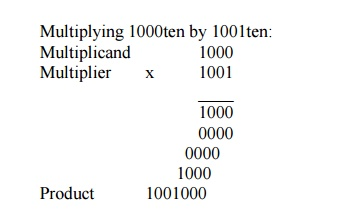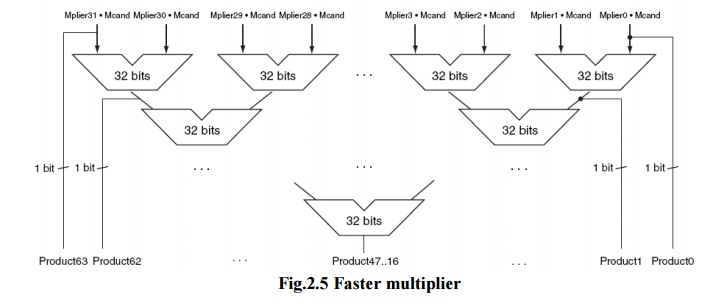Home | | Computer Architecture | Arithmetic Operations: Multiplication

# Arithmetic Operations: Multiplication

The first operand is called the multiplicand and the second the multiplier. The final result is called the product.

MULTIPLICATIONFig. 2.3 First version of the multiplication hardwareThe first operand is called the multiplicand and the second the multiplier. The final result is called the product. As you may recall, the algorithm learned in grammar school is to take the digits of the multiplier one at a time from right to left, multiplying the multiplicand by the single digit of the multiplier and shifting the intermediate product one digit to the left of the earlier intermediate products.

The first observation is that the number of digits in the product is considerably larger than the number in either the multiplicand or the multiplier. In fact, if we ignore the sign bits, the length of the multiplication of an n-bit multiplicand and an m-bit multiplier is a product that is n + m bits long. That is, n + m bits are required to represent all possible products. Hence, like add, multiply must cope with overflow because we frequently want a 32-bit product as the result of multiplying two 32-bit numbers.

In this example we restricted the decimal digits to 0 and 1. With only two choices, each step of the multiplication is simple:

1. Just place a copy of the multiplicand in the proper place if the multiplier digit is a 1, or

2. Place 0 (0 ¥ multiplicand) in the proper place if the digit is 0.

The multiplier is in the 32-bit Multiplier register and that the 64-bit Product register is initialized to 0. Over 32 steps a 32-bit multiplicand would move 32 bits to the left. Hence we need a 64-bit Multiplicand register, initialized with the 32-bit multiplicand in the right half and 0 in the left half. This register is then shifted left 1 bit each step to align the multiplicand with the sum being accumulated in the 64-bit Product register.

Moore’s Law has provided so much more in resources that hardware designers can now build a much faster multiplication hardware. Whether the multiplicand is to be added or not is known at the beginning of the multiplication by looking at each of the 32 multiplier bits. Faster multiplications are possible by essentially providing one 32-bit adder for each bit of the multiplier: one input is the multiplicand ANDed with a multiplier bit and the other is the output of a prior adder.

SIGNED MULTIPLICATION

·        In the signed multiplication, convert the multiplier and multiplicand to positive numbers and then remember the original signs.

·        The algorithms should then be run for 31 iterations, leaving the signs out of the calculation

·        The shifting steps would need to extend the sign of the product for signed numbers. When the algorithm completes, the lower word would have the 32-bit product.

FASTER MULTIPLICATIONFig.2.5 Faster multiplier

·        Faster multiplications are possible by essentially providing one 32-bit adder for each bit of the multiplier: one input is the multiplicand ANDed with a multiplier bit, and the other is the output of a prior adder.

·        Connect the outputs of adders on the right to the inputs of adders on the left, making a stack of adders 32 high.

·        The above figure shows an alternative way to organize 32 additions in a parallel tree. Instead of waiting for 32 add times, we wait just the log2 (32) or five 32-bit add times.

·        Multiply can go even faster than five add times because of the use of carry save adders.

·        It is easy to pipeline such a design to be able to support many multiplies simultaneously

Multiply in MIPS

MIPS provides a separate pair of 32-bit registers to contain the 64-bit product, called Hi and Lo. To produce a properly signed or unsigned product, MIPS has two instructions: multiply (mult) and multiply unsigned (multu). To fetch the integer 32-bit product, the programmer uses move from lo (mflo). The MIPS assembler generates a pseudoinstruction for multiply that specifies three generalpurpose registers, generating mflo and mfhi instructions to place the product into registers.

Booth Algorithm

Booth’s Algorithm Registers and Setup

3 n bit registers, 1 bit register logically to the right of Q (denoted as Q-1 )

Register set up

Q register <- multiplier

Q-1 <- 0

M register <- multiplicand

A register <-

Count <- n

Product will be 2n bits in A Q registers Booth’s Algorithm Control Logic

Bits of the multiplier are scanned one at a a time (the current bit Q0 )

As bit is examined the bit to the right is considered also (the previous bit Q-1 )Fig. 2.6 Booth algrithm

• Then:

00: Middle of a string of 0s, so no arithmetic operation.

01: End of a string of 1s, so add the multiplicand to the left half of the product (A).

10:            Beginning of a string of 1s, so subtract the multiplicand from the left half of the product (A).

11:            Middle of a string of 1s, so no arithmetic operation.

Then shift A, Q, bit Q-1 right one bit using an arithmetic shift

In an arithmetic shift, the msb remainsStudy Material, Lecturing Notes, Assignment, Reference, Wiki description explanation, brief detail
Computer Architecture : Arithmetic Operations : Arithmetic Operations: Multiplication |